Next: IR Optical Depth using Up: Infrared Spectrum Previous: IR Scattering from Mie

### IR Optical Depth Inversion

The radiative transfer solution for a cloudy atmosphere, Equation 6, was derived earlier. A solution for the optical depth of the cloud can now be obtained by inverting Equation 6 for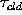in terms of the variables discussed in the previous sections,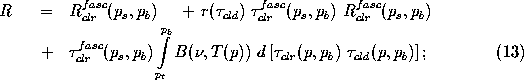where the radiance contribution above the cloud was shown to be negligible by the FASCOD3P model, the upwelling model radiance includes the terrestrial contribution, the model derived radiances are corrected based on Equation 8, and the spectral dependence is implied. Rearranging terms results in a radiance value that effectively projects the instrument to cloud base: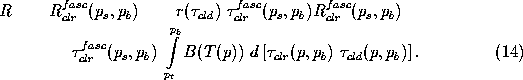Integration by parts transforms the right hand side, such that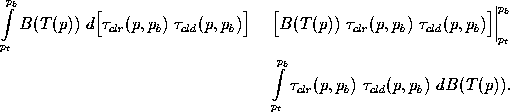The Trapezoid Rule is applied to the remaining integral at the endpoints to obtain a final solution for the cloud transmissivity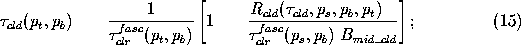where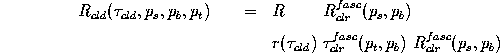is the downwelling cloud radiance; and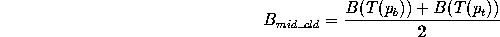is the average Planck radiance of the cloud determined from local radiosonde data and lidar derived cloud boundaries, and the clear sky transmissivity within the cloud,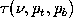, is obtained from FASCOD3P. The solution is iterated forin the reflectance term until a solution converges to within 0.001 (Ackerman et al., 1990).

The algorithm takes into consideration the fact that the cloud may be opaque. This reduces the effective cloud top altitude, while increasing the effective cloud top temperature. Equation 15 would yield an unphysical solution based on true cloud base and top temperatures. Therefore, a direct solution of Equation 15 is not attempted because the measured radiance for an opaque cloud will be larger than the mid-cloud radiance determined from radiosonde measurements. The result is a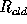: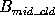ratio that becomes larger than unity, yielding a negative, unphysical solution of Equation 15.

Instead, the cloud is broken into vertical layers defined by the radiosonde temperature profile, where the cloud transmittance is calculated from the cloud base to a given altitude layer. If the transmittance becomes negative, the cloud is labeled as opaque at the previous altitude. Otherwise, the process continues until the cloud top is reached, where the solution is taken to be the cloud infrared transmittance. It will be shown that a cloud transmittance less than 0.05 (infrared optical depth > 3) is the minimum resolvable value.Next: IR Optical Depth using Up: Infrared Spectrum Previous: IR Scattering from Mie

Daniel DeSlover
Sun Aug 11 10:02:40 CDT 1996